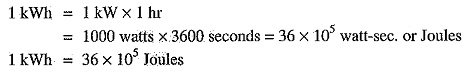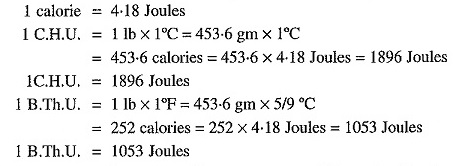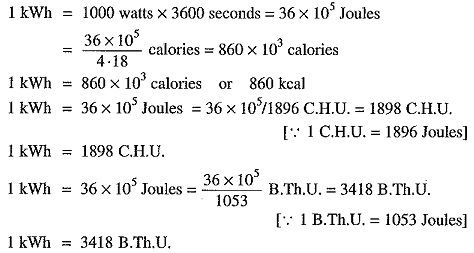## Unit of Energy | Fundamentals of Energy:

The capacity of an agent to do work is known as its energy. The most important forms of Unit of Energy are

• Mechanical energy,
• Electrical energy and
• Thermal energy.

Different units have been assigned to various forms of energy. However, it must be realized that since mechanical, electrical and thermal energies are interchangeable, it is possible to assign the same unit to them.

#### 1. Mechanical energy:

The unit of mechanical energy is newton-metre or joule on the M.K.S. or SI system.

The work done on a body is one newton-metre (or joule) if a force of one newton moves it through a distance of one metre i.e.,

Mechanical energy in joules = Force in newton x distance in meters

#### 2. Electrical energy:

The unit of electrical energy is watt-sec or joule and is defined as follows:

One watt-second (or joule) energy is transferred between two points if a p.d. of 1 volt exists between them and 1 ampere current passes between them for 1 second i.e.,

Electrical energy in watt-sec (or joules) = voltage in volts x current in amperes x time in seconds

Joule or watt-sec is a very small unit of electrical energy for practical purposes. In practice, for the measurement of electrical energy, bigger units viz., watt-hour and kilowatt hour are used.

1 watt-hour =  1 watt x 1 hr

= 1 watt x 3600 sec =  3600 watt-sec

1 kilowatt hour (kWh) = 1 kW x 1 hr = 1000 watt x 3600 sec =  36 x 105 watt-sec.

#### 3. Thermal energy:

Heat is a form of energy which produces the sensation of warmth. The unit of heat is calorie, British thermal unit (B.Th.U.) and centigrade heat units (C.H.U.) on the various systems.

Caloric: It is the amount of heat required to raise the temperature of 1 gm of water through 1°C

i.e.,

1 calorie = 1 gm of water x 1C

Sometimes a bigger unit namely kilocalorie is used. A kilocalorie is the amount of heat required to raise the temperature of 1 kg of water through 1°C i.e.,

1 kilocalorie = 1 kg x 1C = 1000 gm x 1C = 1000 calories

B.Th.U. It is the amount of heat required to raise the temperature of 1 lb of water through 1°F i.e.,

1 B.Th.U. = 1 lb x 1°F

C.H.U. It is the amount of heat required to raise the temperature of I lb of water through 1°C i.e.,

1 C.H.U. = 1 lb x 1°C

#### Relationship Among Energy Units:

The energy whether possessed by an electrical system or mechanical system or thermal system has the same thing in common i.e., it can do some work. Therefore, mechanical, electrical and thermal energies must have the same unit. This is amply established by the fact that there exists a definite relationship among the units assigned to these energies. It will be seen that these units are related to each other by some constant.

##### (i) Electrical and Mechanical:It is clear that electrical energy can be expressed in Joules instead of kWh.

##### (ii) Heat and Mechanical:It may be seen that heat energy can be expressed in Joules instead of thermal units viz. calorie, B.Th.U. and C.H.U.

##### (iii) Electrical and Heat:The reader may note that Unit of Energy can be converted into heat and vice-versa. This is expected since electrical and thermal energies are interchangeable.

Scroll to Top